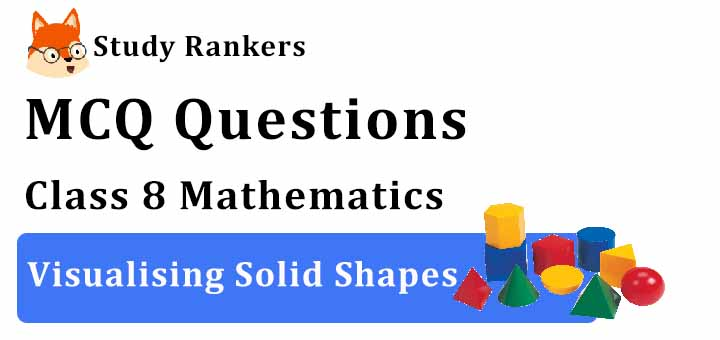## MCQ Questions for Class 8 Maths: Ch 10 Visualising Solid Shapes1. Which is the three-dimensional figure formed by rotating a triangle?
(a) Cone
(c) Prism
(d) Square
► (a) Cone

2. How many congruent edges does a cube have?
(a) 12
(b) 6
(c) 8
(d) none of these
► (a) 12

3. A square pyramid always has ___.
(a) Four lateral faces, which are parallel to each other.
(b) Four lateral faces, which are congruent equilateral triangles and a rectangular base.
(c) Two bases which are congruent and parallel.
(d) Four lateral faces, which are congruent isosceles triangles and a square base.
► (d) Four lateral faces, which are congruent isosceles triangles and a square base.

4. How many circular bases does a cylinder have?
(a) 1
(b) 2
(c) 3
(d) 4
► (b) 2

5. How many vertices does a triangular pyramid have ?
(a) 1
(b) 2
(c) 3
(d) 4
► (d) 4

6. The lateral faces of a pyramid are____.
(a) triangles
(b) pentagons
(c) rectangles
(d) None of these
► (a) triangles

7. How many triangles would you find in a net that folds into a square pyramid?
(a) 3
(b) 4
(c) 5
(d) 6
► (b) 4

8. Identify the statement that is false for a prism.
(a) A right prism has rectangular lateral faces.
(b) A right prism has two bases.
(c) A right prism has triangular lateral faces.
(d) A right prism has identical parallel faces.

9. What is the difference between a rectangle and a cube?
(a) A rectangle is 3-dimensional and a cube is 2-dimensional.
(b) A rectangle and a cube are both 2-dimensional.
(c) A rectangle is 2-dimensional and a cube is 3-dimensional.
(d) A rectangle and a cube are both 3-dimensional.
► (c) A rectangle is 2-dimensional and a cube is 3-dimensional.

10. Which of the following statements is true?
(a) The lateral faces of a square prism are triangles.
(b) The lateral faces of a triangular prism can be squares or rectangles.
(c) The lateral faces of a square pyramid can be squares.
(d) The lateral faces of a triangular pyramid can be squares or rectangles.
► (b) The lateral faces of a triangular prism can be squares or rectangles.

11. How many vertices does a cone have?
(a) 2
(b) 1
(c) 3
(d) 4
► (b) 1

12. For a polyhedron, if ‘F’ stands for number of faces, V stands for number of vertices and E stands for number of edges, then which of the following relationships is named as Euler’s formula ?
(a) F + V = E + 2
(b) F + E = V + 2
(c) V + E = F + 2
(d) F+ V = E – 2
► (a) F + V = E + 2

13. The top-view of a cube looks like a:
(a) Circle
(b) Square
(c) Rectangle
(d) Triangle
► (b) Square

14. The top-view of a cuboid looks like a:
(a) Circle
(b) Square
(c) Rectangle
(d) Triangle
► (c) Rectangle

15. How many circular bases does a cylinder have?
(a) 2
(b) 3
(c) 4
(d) 1
► (a) 2

16. The faces of a triangular pyramid consist of
(a) 1 square and 3 triangles
(b) 2 triangles and 3 rectangles
(c) 4 triangles
(d) 2 rectangles and 3 triangles
► (c) 4 triangles

17. A three dimensional shape is _________ object.
(a) solid
(b) 2d
(c) plane
(d) None of these
► (a) solid

18. What do you call solid figures with line segments as their edges?
(a) Polygons
(b) Squares
(c) Cylinders
(d) Polyhedrons
► (d) Polyhedrons

19. Which of the following can be other name of a cylinder?
(a) A triangular prism
(b) A rectangular prism
(c) A vertical prism
(d) A circular prism
► (d) A circular prism

20. Which is/are three dimensional shapes?
(a) Sphere
(b) Cylinder
(c) Cone
(d) All of the above
► (d) All of the above

21. Which of the following statements is false?
(a) A sphere has one flat surface.
(b) A cone has one flat face.
(c) A cylinder has two circular faces.
(d) A sphere has one curved face.
► (a) A sphere has one flat surface.

22. The faces of a cube consist of
(a) 8 squares
(b) 4 squares and 2 rectangles
(c) 2 squares and 4 rectangle
(d) 6 squares
► (d) 6 squares